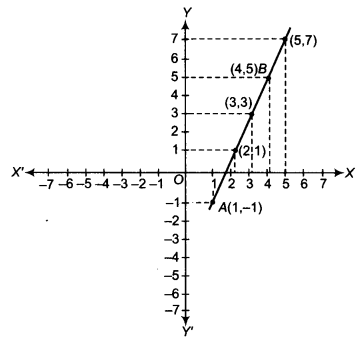# Plot the points A (1, -1) and B (4,5)

Plot the points A (1, -1) and B (4,5).
(i) Draw the line segment joining these points. Write the coordinates of a point on this line segment between the points A and B.
(ii) Extend this line segment and write the coordinates of a point on this line which lies outside the line segment AB.

Firstly, draw two perpendicular axes and marks the points on the axes. Now plot the points A(1, -1) and B (4, 5) on a graph paper and join them to get the line AB(i) The coordinate of a point on this line segment
between the points A and B is (2, 1). Point (3, 3) also lies on this line segment.
(ii) The coordinates of a point on this line which lies outside the line segment AB is (5, 7).